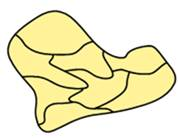# Map Coloring A fictional map of the countries of a continent is given. Represent the map by a graph and find a coloring of the graph that uses the fewest possible number of colors. Then color the map according to the graph coloring you found.### Mathematical Excursions (MindTap C...

4th Edition
Richard N. Aufmann + 3 others
Publisher: Cengage Learning
ISBN: 9781305965584

#### Solutions

Chapter
Section### Mathematical Excursions (MindTap C...

4th Edition
Richard N. Aufmann + 3 others
Publisher: Cengage Learning
ISBN: 9781305965584
Chapter 5.4, Problem 3ES
Textbook Problem
26 views

## Map Coloring A fictional map of the countries of a continent is given. Represent the map by a graph and find a coloring of the graph that uses the fewest possible number of colors. Then color the map according to the graph coloring you found.To determine

The fewest possible number of colours to colour the map using graph colouring.

### Explanation of Solution

Given information:

A fictional map of the countries of the continent is

Name the given graph as follows:

The neighbouring countries for each country are:

• A: B, F
• B: A, F, E, C
• C: B, E, D
• D: C, E, H
• E: C, B, F, G, H, D
• F: A, B, E, G
• G: F, E, H
• H: G, E, D

The graph is given by

### Still sussing out bartleby?

Check out a sample textbook solution.

See a sample solution

#### The Solution to Your Study Problems

Bartleby provides explanations to thousands of textbook problems written by our experts, many with advanced degrees!

Get Started

Find more solutions based on key concepts
In Exercises 75-98, perform the indicated operations and/or simplify each expression. 89. (x + 2y)2

Applied Calculus for the Managerial, Life, and Social Sciences: A Brief Approach

Factor completely: 42x413x240

Elementary Technical Mathematics

Divide the following fractions and reduce to lowest terms. 27.

Contemporary Mathematics for Business & Consumers

Solve each inequality. 9+16

Trigonometry (MindTap Course List)

Change each decimal into a common fraction. 0.17-

College Algebra (MindTap Course List)

The general solution to (for x, y > 0) is: a) y = ln x + C b) c) y = ln(ln x + C) d)

Study Guide for Stewart's Single Variable Calculus: Early Transcendentals, 8th

In Problems 1926 solve the given differential equation. 19. (y2 + 1) dx = y sec2 x dy

A First Course in Differential Equations with Modeling Applications (MindTap Course List)

54. The time (in minutes) between telephone calls at an insurance claims office has the following exponential p...

Modern Business Statistics with Microsoft Office Excel (with XLSTAT Education Edition Printed Access Card) (MindTap Course List)

Counting when Order Matters The factorial function occurs often in probability and statistics. For a non-negati...

Functions and Change: A Modeling Approach to College Algebra (MindTap Course List)